Hex-Switch Decoder Uses Weighted-Capacitor Network to Reduce I/O Pin Count

Texas Instruments CD40106B

Ajoy Raman

Electronic Design

By using this simple capacitor-network circuit and software, designs using the Arduino Uno need only two I/O pins to read a hex-switch decoder, leaving the remaining pins for other functions.

A pulse-width-modulated (PWM) signal and the RC-charging characteristics of a weighted-capacitor network can be used along with a CMOS Schmitt inverter to generate a pulse whose width is linearly proportional to the 16 combinations of a hex (thumbwheel) switch. The decoding is implemented using the Arduino Uno and requires only two pins (OCRA and INT0), leaving all of the other pins free for alternate functions.

The theory, which is the basis for this design idea, is the well-known capacitor-charging equation: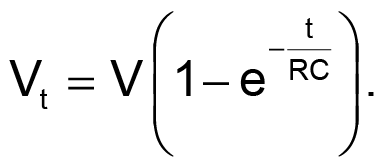Time T required to reach a voltage k × V is given by: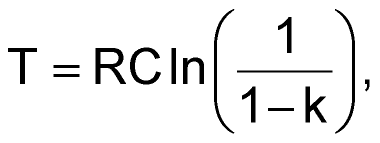which shows a linear relationship with C for a fixed value of R.

PWM output OCRA of the Arduino Uno forms the input to the circuit (Fig. 1), with the output connected to the interrupt pin INT0.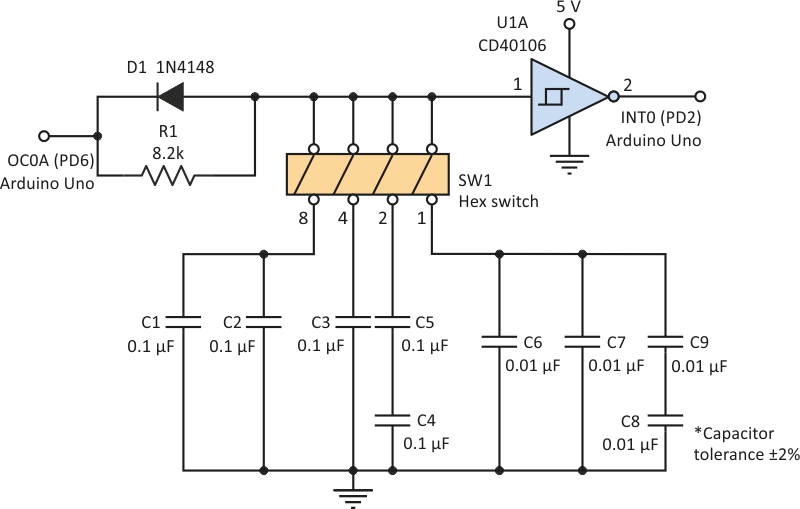Figure 1. The functional block diagram consists of a hex switch connected to the weighted-capacitor network, charging resistor R1, discharge diode D1, and the Schmitt inverter.

Capacitors in the ratio of 8:4:2:1 are selected by SW1, then charged through R1 during the high-state portion of the PWM signal and discharged through diode D1 on the low-state. At the start of the charge cycle, the output of the Schmitt inverter, U1A, is high; it changes to low when the capacitor voltage reaches the upper threshold of the Schmitt inverter. This negative-going edge is used as an interrupt to the processor.

The Arduino Uno, operating with a 16-MHz clock, is programmed to use Timer0 to set up the PWM output OCRA at 244 Hz with the pulse-width set to 224/255 cycles. Figure 2 shows the waveforms when only switch #8 of the SW1 is ON. Parallel capacitors C1 and C2 are charged by the high-state output V-high of OCRA, and the Schmitt inverter changes state at the input upper-threshold of approximately two-thirds the supply voltage. This negative transition uses INT0 to interrupt the processor, which then reads the current value of Timer0.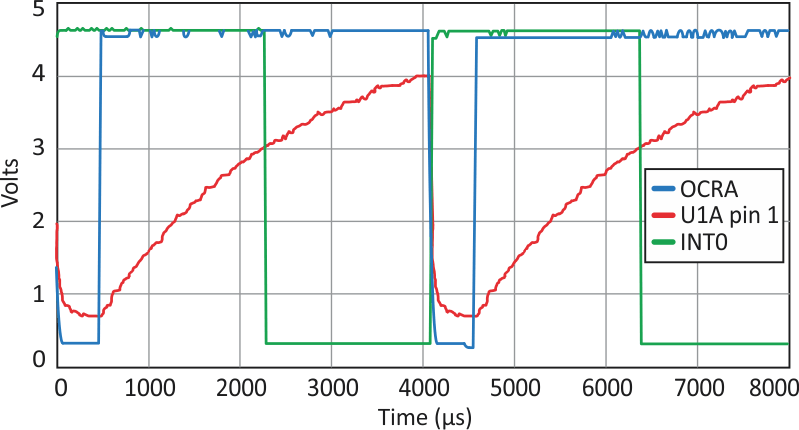Figure 2. The Arduino PWM output OCRA charges the weighted-capacitor network, generating INT0 at the Schmitt trigger’s upper threshold of approximately 3 V.

By approximating the value of V-high to be the same as the supply voltage allows pulse-width T to be derived using the formula: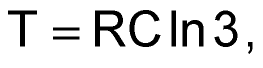which is independent of the supply voltage. With RC values of R = 8.2 kΩ and C= 0.2 µF, T is 1801 µs of a PWM period of 4096 µs, giving a Timer0 count of 112. The experimental value obtained is 115.

The Table 1 shows the Timer0 count captured by the interrupts for the 16-combinations of SW1. The count values at different supply voltages are also shown.

Table 1. Experimental values of Timer0 count with SW1 settings
 SW1 Setting 0 1 2 3 4 5 6 7 8 9 10 11 12 13 14 15 VCC 4.5 V 0 15 31 45 60 73 88 102 115 129 143 157 171 184 199 212 VCC 4.0 V 0 15 31 44 59 73 88 101 115 128 142 155 169 182 197 210 VCC 5.0 V 0 14 30 44 58 72 87 100 113 127 142 155 169 182 197 210

As shown in Figure 3, the count values captured at the different interrupts corresponding to the 16 combinations of SW1 reveal a fairly linear characteristic, with a count difference of about 14 between settings. A software routine that permits a maximum-tolerable difference of ±6 with respect to nominal mid-values is used to decode the switch positions.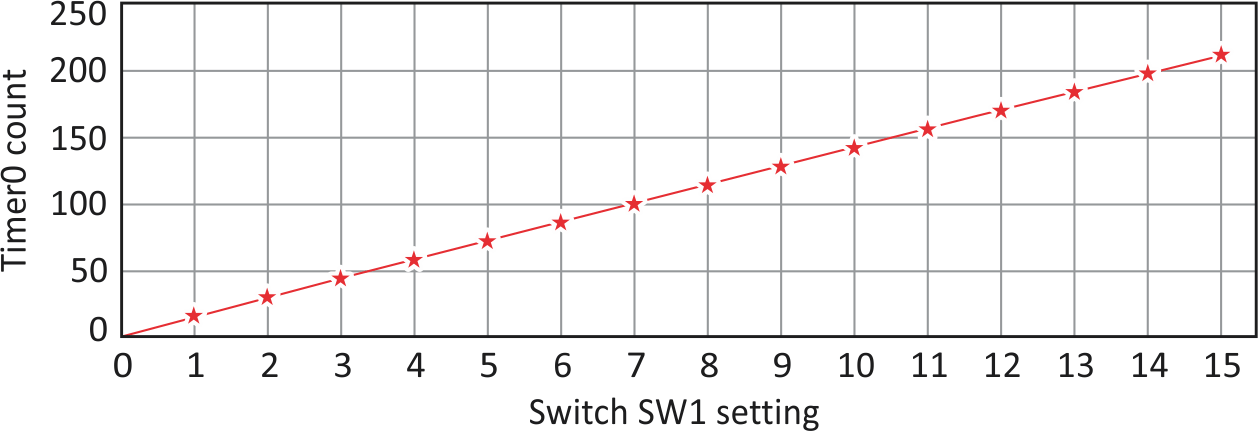Figure 3. The count values seen at the different interrupts that match the 16 combinations of SW1 show a good linearity of the result.

Figure 4 demonstrates that as both V-high of OCRA and the upper threshold of the Schmitt-inverter input vary proportionately with supply voltage, the change in the Timer0 count captured by the interrupts for the 16 combinations of SW1 are within acceptable limits. This is shown with respect to the values at 4.5 V, with the circuit operation at 4.0 V and 5.0 V.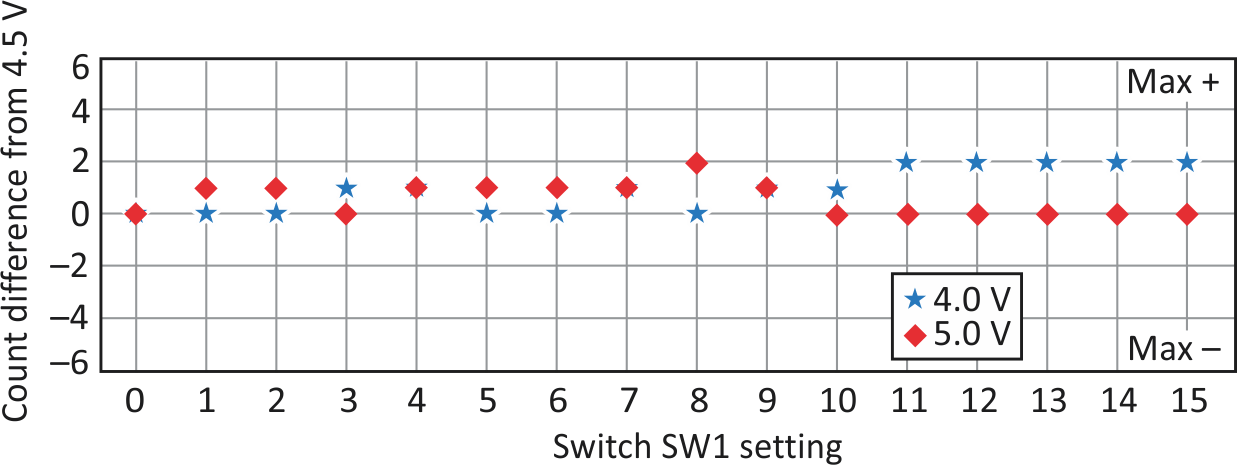Figure 4. The plot of the count difference versus supply voltage demonstrates that changes for the 16 combinations of SW1 in the Timer0 count, as seen by the interrupts, are within acceptable limits.

Listing 1 shows the C code block used to decode the switch setting. A variable “duty” corresponding to the Timer0 count is passed to the function “get-switch,” which then returns the switch values based on comparing the count with the integer mid-values of the nominal count.

Listing 1. This block of C code decodes the Timer0 count and determines the switch value based on that count.

get_switch(uint8_t duty){
if (duty<=7){ switch_value=00;}
else{if (duty> 7 && duty <=22){switch_value=l;}

else{if (duty> 22 && duty <=37){switch_value=2;}
else{if (duty> 37 && duty <=51){switch_value=3;}
else{if (duty> 51 && duty <=66){switch_value=4;}
else{if (duty> 66 && duty <=80){switch_value=5;}
else{if (duty> 80 && duty <=95){switch_value=6;}
else{if (duty> 95 && duty <=108){switch_value=7;}
else{if (duty> 108 && duty <=122){switch_value=8;}
else{if (duty> 122 && duty <=136){switch_value=9;}
else{if (duty> 136 && duty <=150){switch_value=10;}
else{if (duty> 150 && duty <=164){switch_value=ll;}
else{if (duty> 164 && duty <=177){switch_value=12;}
else{if  (duty> 177 && duty <=191){switch_value=13;}
else{if (duty> 191 && duty <=205){switch_value=14;}
else{if (duty> 205){switch_value=15;}

}}}}}}}}}}}}}}}
return (switch_value);
}

Using a common-output OCRA, this method could be expanded to use additional hex switches and the other interrupts available on the Arduino Uno. The advantage of this approach is that it frees up analog-to-digital converters, Rx/Tx, and comparators, and can be used on minimum pin-count microcontrollers.

Materials on the topic

electronicdesign.com

You may have to register before you can post comments and get full access to forum.
 User Name Remember Me? Password